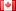# Revision history [back]

### Using numerical solution from system of equations

I want to take the numerical solution of a variable in a system of equations and use it later in the program. But all I can get is the symbolic definition. Here's a simplified example of the problem.

sage: var('x y z')

sage: eq1 = x + y + z == 6

sage: eq2 = 2x - y + 2z == 6

sage: eq3 = 3x + 3y - z == 6

sage: solve([eq1, eq2, eq3], x, y, z)

sage: v = x

sage: print v

Output: [ [x == 1, y == 2, z == 3] ] x

I assume the syntax for solving the system is correct because I get the right answers but I want v = 1, not v = x. Thanks.2 indent code block

### Using numerical solution from system of equations

I want to take the numerical solution of a variable in a system of equations and use it later in the program. But all I can get is the symbolic definition. Here's a simplified example of the problem.

sage: var('x y z')z')
sage: eq1 = x + y + z == 66
sage: eq2 = 2x 2*x - y + 2z 2*z == 66
sage: eq3 = 3x 3*x + 3y 3*y - z == 66
sage: solve([eq1, eq2, eq3], x, y, z)z)

sage: v = x

sage: print v


Output:

sage: v = x

sage: print v

Output:

[
[x == 1, y == 2, z == 3]
]
xx


I assume the syntax for solving the system is correct because I get the right answers but I want v = 1, not v = x. Thanks.3 retaggedDSM5242 ●21 ●68 ●112

### Using numerical solution from system of equations

I want to take the numerical solution of a variable in a system of equations and use it later in the program. But all I can get is the symbolic definition. Here's a simplified example of the problem.

sage: var('x y z')

sage: eq1 = x + y + z == 6

sage: eq2 = 2*x - y + 2*z == 6

sage: eq3 = 3*x + 3*y - z == 6

sage: solve([eq1, eq2, eq3], x, y, z)

sage: v = x

sage: print v


Output:

[
[x == 1, y == 2, z == 3]
]
x


I assume the syntax for solving the system is correct because I get the right answers but I want v = 1, not v = x. Thanks.